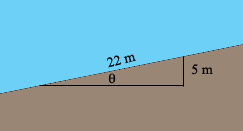SEARCH HOMEMath Central Quandaries & QueriesQuestion from abeth, a teacher: a mountain road drops 5 m for every 22 m of road. Calculate the angle at which the road is inclined to the horizontal to the nearest degree.Hi Abeth,I drew horizontal and vertical line segments to complete a right triangle. I let θ be the measure of the angle of incline. You know the length of the side opposite θ and the length of the hypotenuse. What trig function related the length of the opposite side and the hypotenuse? Use the inverse of this function to find the measure of θ.

PennyMath Central is supported by the University of Regina and The Pacific Institute for the Mathematical Sciences.